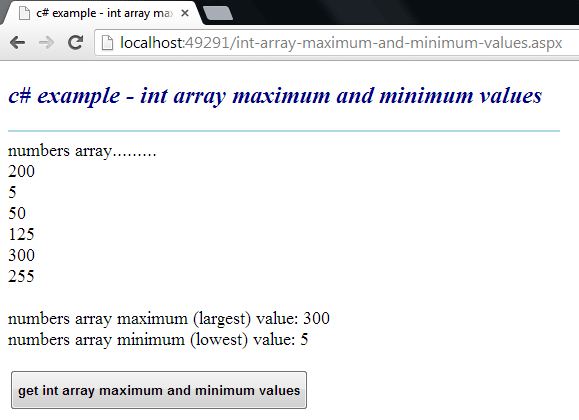### c# - How to get maximum and minimum values from an int array

Int array maximum and minimum value
The following asp.net c# example code demonstrate us how can we get maximum and minimum value from an int array elements programmatically at run time in an asp.net application. .Net framework's int array represent an array of integer (Int32) values.

Int array contains all elements value are integer number, so we can get maximum and minimum value from them. Liq Max() method allow us to get the maximum value from a numeric collection and Min() method return the minimum value from a numeric elements collection.

We can call Max() method for an int array as this way IntArray.Max() and Min method as this way IntArray.Min(). Both method have no required or optional parameter. We just need to call them by specifying a numeric collection.
int-array-maximum-and-minimum-values.aspx
``````
<%@ Page Language="C#" AutoEventWireup="true"%>

<!DOCTYPE html>
<script runat="server">
protected void Button1_Click(object sender, System.EventArgs e)
{
int[] numbers = { 200,5,50,125,300,255};

Label1.Text = "numbers array.........<br />";
foreach (int i in numbers)
{
Label1.Text += i.ToString() + "<br />";
}

int maximumValue = numbers.Max();
int minimumValue = numbers.Min();

Label1.Text += "<br />numbers array maximum (largest) value: " + maximumValue;
Label1.Text += "<br />numbers array minimum (lowest) value: " + minimumValue;
}
</script>

<html xmlns="http://www.w3.org/1999/xhtml">
<title>c# example - int array maximum and minimum values</title>
<body>
<form id="form1" runat="server">
<div>
<h2 style="color:DarkBlue; font-style:italic;">
c# example - int array maximum and minimum values
</h2>
<hr width="550" align="left" color="LightBlue" />

<asp:Label
ID="Label1"
runat="server"
Font-Size="Large"
>
</asp:Label>
<br /><br />
<asp:Button
ID="Button1"
runat="server"
Text="get int array maximum and minimum values"
OnClick="Button1_Click"
Height="40"
Font-Bold="true"
/>
</div>
</form>
</body>
</html>
``````More c# examples